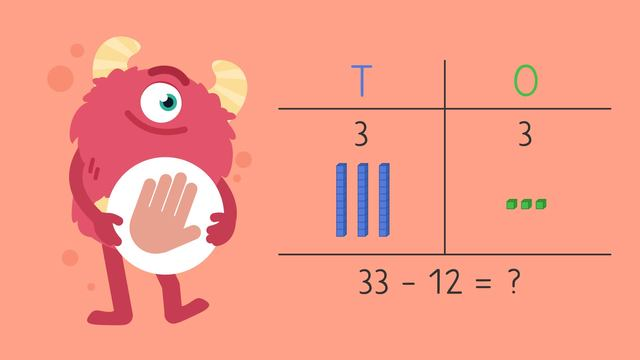# Using Place Value to Subtract Two Digit Numbers (No Regrouping)—Let's PracticeRating

Be the first to give a rating!
The authorsTeam Digital
Using Place Value to Subtract Two Digit Numbers (No Regrouping)—Let's Practice
CCSS.MATH.CONTENT.2.NBT.B.5

### TranscriptUsing Place Value to Subtract Two Digit Numbers (No Regrouping)—Let's Practice

Razzi says get these items ready (...) Because today we're going to practice... Using Place Value to Subtract (no regrouping). It's time to begin! Let's use base ten blocks to subtract. What is thirty-three minus twelve? We can represent thirty-three with three tens and three ones. Then cross off two ones and one ten... which leaves twenty-one. Did you also get twenty-one? Let's tackle the next problem! What is fifty-eight minus twenty-eight? We can represent fifty-eight with five tens and eight ones. Then cross off eight ones and two tens... which leaves thirty. Did you also get thirty? Let's try one more! What is eighty-three minus forty-two? We can represent eighty-three with eight tens and three ones. Then cross off two ones and four tens... which leaves forty-one. Did you also get forty-one? Razzi had so much fun practicing with you today! See you next time!